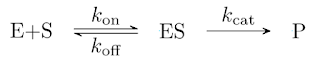A fully suicidal enzyme follows a second order equation

## Saturday 4 August 2018

### A fully suicidal enzyme follows a second order equation

That a fully suicidal enzyme follows a second order equation is a rather intuitive statement, but it is nice to have formal proof, which is as follows.

To be specific, a suicide reaction is a reaction that results in enzyme inactivation. This can be full or partial, as is the case of reactions whose product is reactive and that sometimes reacts as is the case with alanine racemase and chloroalanine. Amusingly, a FAD enzyme in vitro with no FAD regeneration is a suicide enzyme.
A second order reaction is one where two substrates enter and one or more come out that do not interact with the substrates. A normal enzyme under Michaelis–Menten kinetics will go back and react with a substrate over and over.
Given a reaction as follows:This does not follow Michaelis–Menten kinetics as the rate of E is: $$\frac{d[E]}{dt} = [ES]k_{\text{off}} - [E][S]k_{\text{on}}$$ Confer $$\frac{d[E]}{dt} = [ES]\cdot k_{\text{off}} + [ES]\cdot k_{\text{cat}} - [E]\cdot [S]\cdot k_{\text{on}}$$ The rate of [ES] is the same, as is the steady state assumption. However [E] does not get decomposed as it depends on [P]. As a result $$\frac{d[P]}{dt} = \frac{k_{\text{on}}}{\frac{k_{\text{off}}}{k_{\text{cat}}}+1}[E][S]$$ Which is the same as a 2nd order. Therefore the latter is the correct model.